# Euclid : Book 1 : Definition 10

The Elements of Euclid - Part 1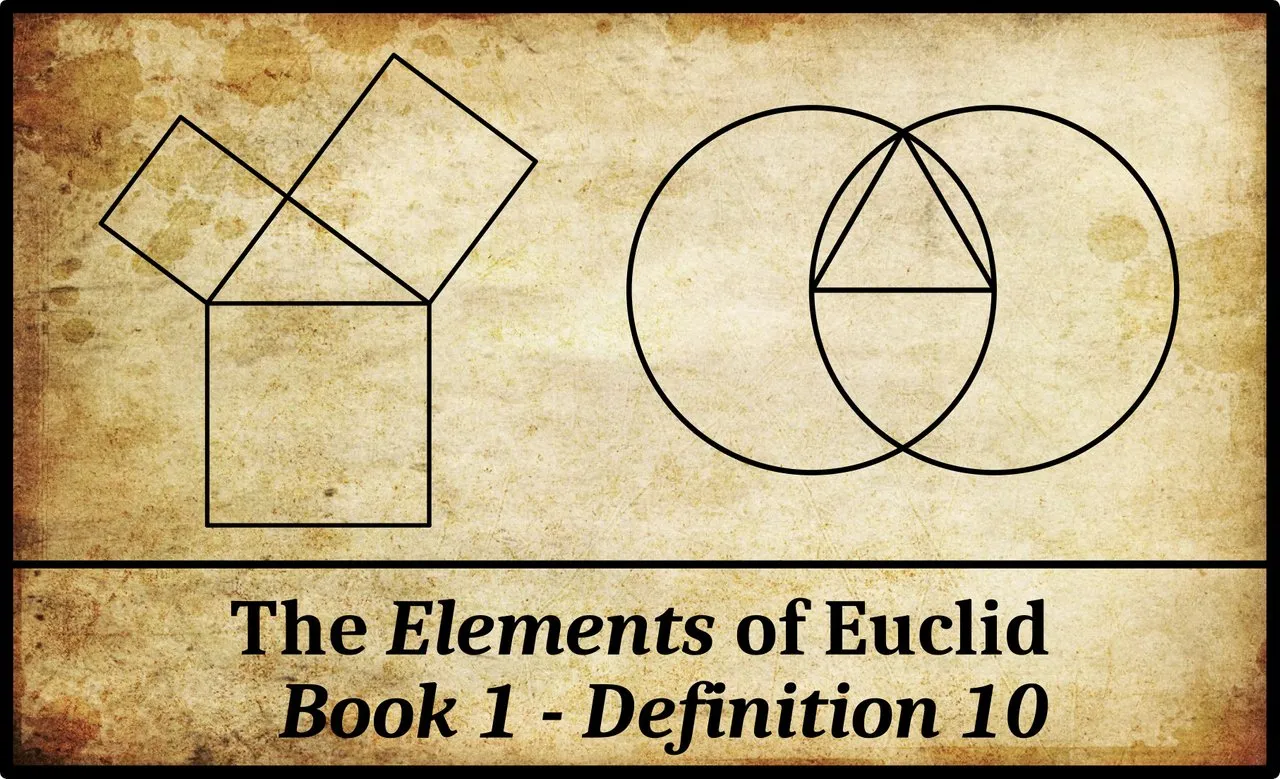In Book 1 of Euclid’s Elements, Definition 10 reads (Fitzpatrick 6):

GreekEnglish
ιʹ. ̔́Οταν δὲ εὐθεῖα ἐπ ̓ εὐθεῖαν σταθεῖσα τὰς ἐφεξῆς γωνίας ἴσας ἀλλήλαις ποιῇ, ὀρθὴ ἑκατέρα τῶν ἴσων γωνιῶν ἐστι, καὶ ἡ ἐφεστηκυῖα εὐθεῖα κάθετος καλεῖται, ἐφ ̓ ἣν ἐφέστηκεν.10. And when a straight-line stood upon (another) straight-line makes adjacent angles (which are) equal to one another, each of the equal angles is a right-angle, and the former straight-line is called a perpendicular to that upon which it stands.

# Magnitudes and Measurements

In the preceding article in this series, we saw that Euclid makes a distinction between the magnitude of a rectilinear angle and its measurement. The latter is characterized by a number, but the magnitude of an angle is, as it were, a pre-numerate concept. In terms of magnitudes, one angle may be greater than, less than, or equal to another angle. In other words, angular magnitudes can be compared to one another, but without the metrical concept of numerical measurement we cannot be more specific than this. We cannot say, for example, that one angle is twice as big as another angle, or half as big.

In Euclid, the magnitudes of rectilinear angles can even be added together, though this addition is very different from arithmetic addition. For example, in Proposition 1:32, Euclid proves a familiar theorem: In any triangle, if one of the sides is produced then the external angle is equal to the sum of the two internal and opposite angles. He does not say anything about the measurements of these angles, simply that the magnitude of the external angle is equal to the magnitude one would get if one were to combine the two internal opposite angles into a single continuous angle.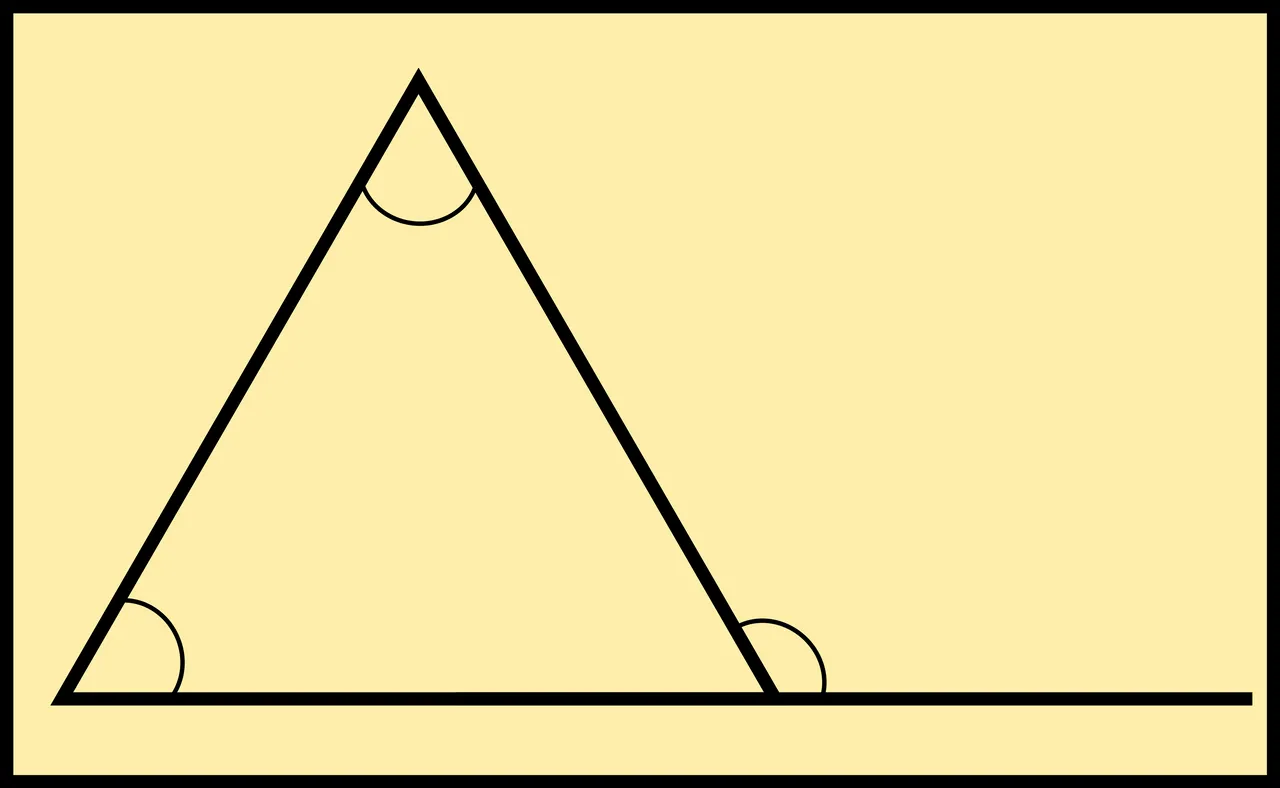The Elements: Proposition 1:32

The arithmetic of angular magnitudes is introduced by Euclid in Proposition 1:13. We will revisit this concept when we reach that proposition.

In the Elements, the only rectilinear angles whose numerical measurements are considered are the right-angle and the straight angle. In Euclid’s day, metrical units for measuring angles—such as degrees and radians—had not yet been introduced. Instead, Euclid defines right-angle as the metrical unit for measuring the rectilinear angle between two lines that are perpendicular to each other. This is, in fact, the purpose of Definition 10. He later uses the metrical unit two right-angles to measure straight angles (eg Propositions 1:13-15).

Thus, in Proposition 1:32, where Euclid also demonstrates that the sum of the three internal angles of a triangle is 180°, he expresses this by saying: the three internal angles of the triangle are equal to two right-angles. This is a numerical equation: the sum of three numbers equals another number. But it also implies that the “sum” of the magnitudes of the three internal angles is equal to the magnitude of a straight angle.

Definition 10, then, defines ὀρθὴ γωνία [orthē gōnia], or right-angle, as the metrical unit for measuring the rectilinear angle between two lines that are perpendicular to each other. It also defines the term κάθετος [kathetos], or perpendicular, in terms of the equality of the magnitudes of the adjacent angles when two lines cross.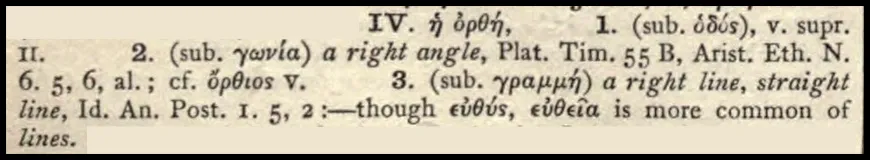ὀρθὴ (Liddell & Scott 1072)

Note that the feminine adjective ὀρθὴ can also be used alone as a substantive to designate a right-angle—just as the feminine adjective εὐθεῖᾰ, straight, can be used as a noun, meaning straight line. Aristotle, for instance, uses ὀρθὴ in this sense in the Nicomachean Ethics:

For a carpenter and a geometer look for right angles in different ways; the former does so in so far as the right angle is useful for his work, while the latter inquires what it is or what sort of thing it is; for he is a spectator of the truth. (Barnes 3732)

καὶ γὰρ τέκτων καὶ γεωμέτρης διαφερόντως ἐπιζητοῦσι τὴν ὀρθήν: ὃ μὲν γὰρ ἐφ᾽ ὅσον χρησίμη πρὸς τὸ ἔργον, ὃ δὲ τί ἐστιν ἢ ποῖόν τι: θεατὴς γὰρ τἀληθοῦς. τὸν αὐτὸν δὴ τρόπον καὶ ἐν τοῖς ἄλλοις ποιητέον, ὅπως μὴ τὰ πάρεργα τῶν ἔργων πλείω γίνηται. (Nicomachean Ethics 1098a29 ff)

Richard Fitzpatrick parses Euclid’s ὀρθὴ as a noun when he translates Definition 10, whereas Thomas Heath parses it as an adjective:

[Definition] 10. When a straight line set up on a straight line makes the adjacent angles equal is one another, each of the equal angles is right, and the straight line standing on the other is called a perpendicular to that on which it stands. (Heath 1956:181)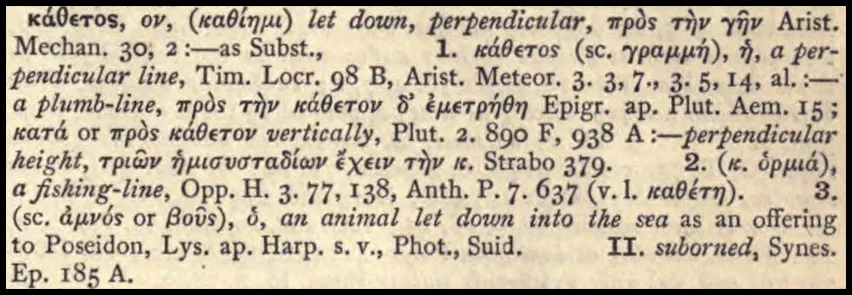κάθετος (Liddell & Scott 721)

Both translators, however, parse κάθετος as a substantive (noun). Like ὀρθὴ, this word can be used as both an adjective and a noun. When used as a noun, it is masculine. The ancient Greek word for line is feminine: (εὐθεῖα [eutheia]). But κάθετος is one of those adjectives whose masculine and feminine forms are the same. Later, in Proposition 1:12, Euclid writes κάθετον εὐθεῖαν γραμμὴν [katheton eutheian grammēn], or perpendicular straight line. The literal meaning of κάθετος is let fall, like a plumb-line, which hangs perpendicular to the ground (Heath 1956:181).

# Proclus and Oenopides

In his Commentary on the First Book of Euclid’s Elements, Proclus tells us that the concept of perpendicularity was also expressed in ancient times as κατὰ γνώμονα [kata gnōmona]: gnomon-wise, like a gnomon, in the manner of a gnomon. A gnomon is an object like a pillar or rod that is used to tell time by the shadow it casts when the sun shines on it. The pointer on a sundial is a gnomon, but the simplest and most primitive species of gnomon is a stick stuck into the ground at right angles to the horizon.

In his discussion of Proposition 1:12—given a line and a point not on the line, to draw a line through the point perpendicular to the given line—Proclus writes:

This problem was first investigated by Oenopides, who thought it useful in astronomy. In archaic fashion, however, he calls the perpendicular a line drawn “gnomonwise,” because the gnomon also is at right angles to the horizon. (Morrow 220-221)

Oenopides of Chios was a 5th-century geometer and astronomer. He was born around 490 BCE on the Ionian island of Chios, which lay off the western coast of Anatolia, about 100 km west of Smyrna (now Izmir). He is said by Eudemus of Rhodes to have been the first person to measure the inclination of the Earth’s axis—or, as he might have expressed it, the angle between the plane of the celestial equator and the ecliptic. This, however, has been disputed by Thomas Heath (Heath 1921:174-175).

The little we know of his contributions to geometry we owe to Proclus. Heath has surmised that Oenopides may be the source of one of the fundamental canons of Greek geometry:

It may therefore be that Oenopides’s significance lay in improvements of method from the point of view of theory; he may, for example, have been the first to lay down the restriction of the means permissible in constructions to the ruler and compasses which became a canon of Greek geometry for all “plane” constructions, i.e. for all problems involving the equivalent of the solution of algebraical equations of degree not higher than the second. (Heath 1921:175-176)

As we saw in an earlier article, Euclidean geometry has often been described as the geometry of the straightedge and compass, although neither of these instruments is ever mentioned by Euclid.

Oenopides is thought to have died around 420 BCE, several decades before Euclid was born. The dates of his birth and death, however, are no more than educated guesses. Proclus tells us that Oenopides was a little younger than Anaxagoras (Morrow 53), and this agrees with other early references to him (Bodnár 17-21).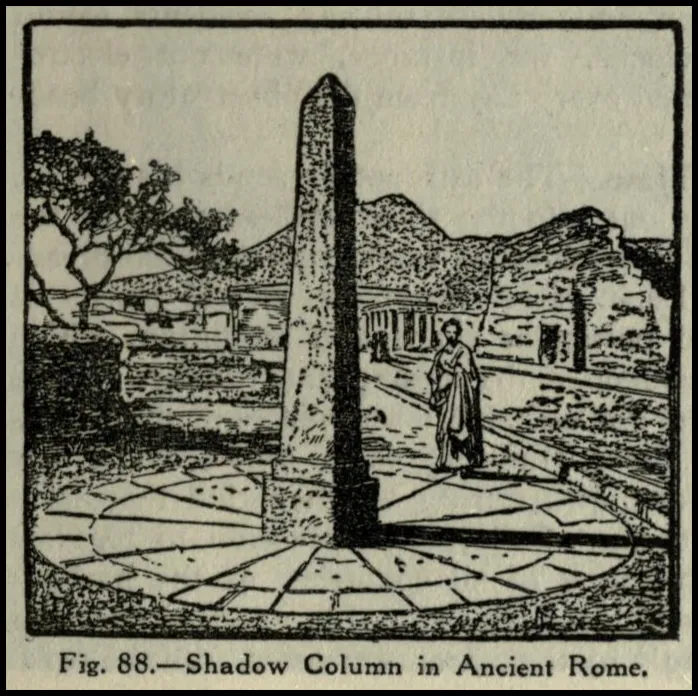A Primitive Gnomon (Bürgel 67)

And that’s a good place to stop.

## Image Credits

• A Primitive Gnomon, Bruno Hans Bürgel, Stella Bloch (translator), Astronomy for All, Page 67, Cassell and Company, Ltd, London (1911):

## Online Resources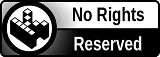H2
H3
H4
3 columns
2 columns
1 column
Join the conversion now